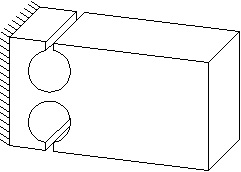Select Page

The flexure hinge is a tried and tested construction element in precision engineering systems. It is created by drilling or spark erosion of two adjacent holes.The positive properties of flexure hinges, free of play and friction, are partly undone by the limited angular rotation. In order to properly dimension a flexure hinge, an optimum must be found between the desired angular rotation, stiffness and maximum material stress. It often requires some calculation until a satisfactory dimensioning has been found. Different formulas are available to achieve this. They are based on theoretically solving force equations or on approximations of finite element analysis (FE) results.

## Theoretical Stiffness Calculation

Calculating the stiffness of a flexure hinge and the material stress that occurs when loaded, is an altogether complex matter. During the course of the years, many articles have been published on solving force equations applicable to the flexure hinge. The article by J.M Paros and L. Weisbord (How to design flexure hinges, Machine Design 25 November 1965) is among the best known ones. N. Lobontiu, Wu and Zhou, and Tseytlin also derived formulas for calculating flexure hinges.

A restriction in these theoretic approaches is that the flexure hinge is analyzed while isolated, meaning that any distortions in the base material next to the contracted part of the flexure hinge are not included. For the design engineer this means that the stiffness of the flexure hinge will be lower in practice.

## Practical Approach by Using FE-Analysis

An accurate calculation of flexure hinge stiffness can be important for the purpose of practical applications. The lecture notes ‘Predicting dynamic behavior and positioning accuracy of constructions and mechanisms‘, W. van der Hoek 1981″ provides empirical equations (based on FE-analysis) for calculating stiffness and material stress values. An article by Y.K. Yong (Review of circular flexure hinge design equations and derivation of empirical formulations, 2007) compares various theoretical and empirical approximations. In this article, he refers to an article by W.O. Schotborgh et all in which the empirical formulas of W. van der Hoek are used. The conclusion by Yong is that these empirical formulas produce the highest accuracy, having an error margin of <2.5%. Since these formulas are easy to apply at the same time, they are very practical to a design engineer.

The FlexHinge freeware, which was developed by Vink System Design & Analysis and which you can download and use for free from this website after registration, uses these empirical equations.